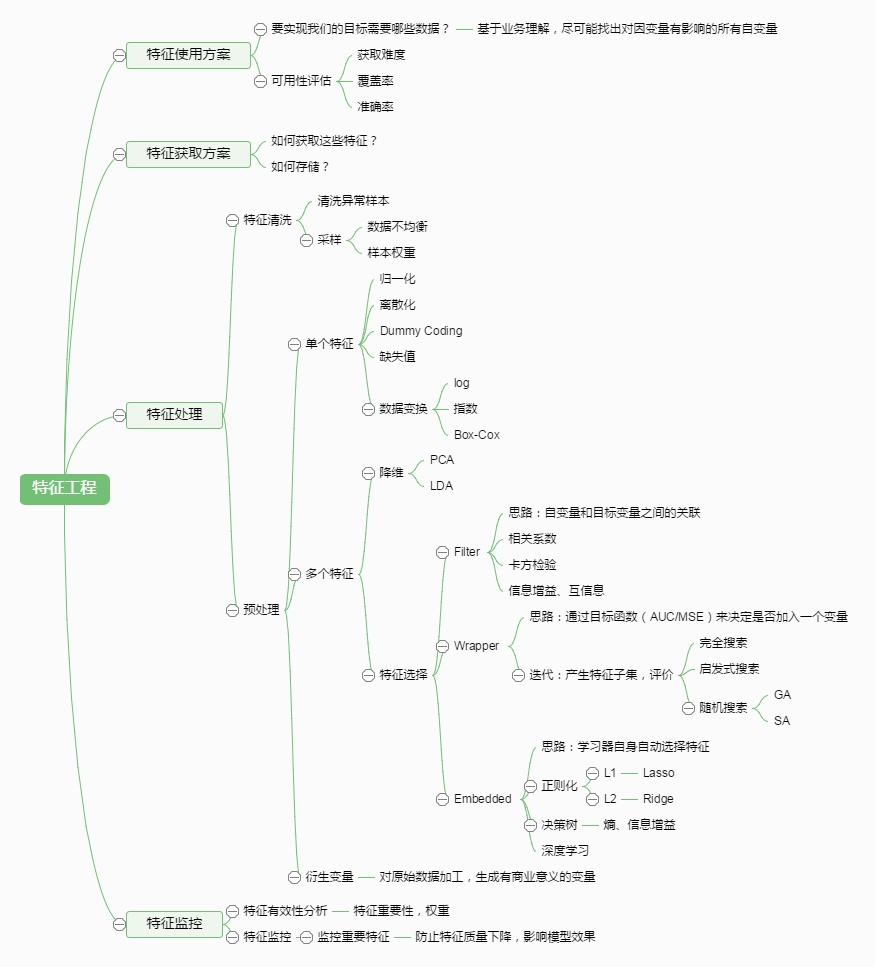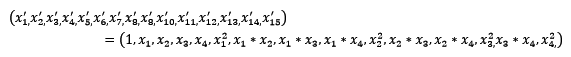# sklearn 特征工程from sklearn.datasets import load_iris

# 导入IRIS数据集

# 特征矩阵
print(iris.data)

# 目标向量
print(iris.target)

## 数据预处理

• 不属于同一量纲：即特征的规格不一样，不能够放在一起比较。无量纲化可以解决这一问题。
• 信息冗余：对于某些定量特征，其包含的有效信息为区间划分，例如学习成绩，假若只关心“及格”或不“及格”，那么需要将定量的考分，转换成“1”和“0”表示及格和未及格。二值化可以解决这一问题。
• 定性（类别）特征不能直接使用：某些机器学习算法和模型只能接受定量特征的输入，那么需要将定性特征转换为定量特征。最简单的方式是为每一种定性值指定一个定量值，但是这种方式过于灵活，增加了调参的工作。通常使用哑编码的方式将定性特征转换为定量特征：假设有N种定性值，则将这一个特征扩展为N种特征，当原始特征值为第i种定性值时，第i个扩展特征赋值为1，其他扩展特征赋值为0。哑编码的方式相比直接指定的方式，不用增加调参的工作，对于线性模型来说，使用哑编码后的特征可达到非线性的效果。
• 存在缺失值：缺失值需要补充。
• 信息利用率低：不同的机器学习算法和模型对数据中信息的利用是不同的，之前提到在线性模型中，使用对定性特征哑编码可以达到非线性的效果。类似地，对定量变量多项式化，或者进行其他的转换，都能达到非线性的效果。

### 无量纲化

#### 标准化

from sklearn.preprocessing import StandardScaler

# 标准化，返回值为标准化后的数据
StandardScaler().fit_transform(iris.data)

#### 区间缩放法

from sklearn.preprocessing import MinMaxScaler

# 区间缩放，返回值为缩放到[0, 1]区间的数据
MinMaxScaler().fit_transform(iris.data)

#### 归一化

from sklearn.preprocessing import Normalizer

# 归一化，返回值为归一化后的数据
Normalizer().fit_transform(iris.data)

### 对定量特征二值化

from sklearn.preprocessing import Binarizer

# 二值化，阈值设置为3，返回值为二值化后的数据
Binarizer(threshold=3).fit_transform(iris.data)

### 对定性特征哑编码

from sklearn.preprocessing import OneHotEncoder

# 哑编码，对 IRIS 数据集的目标值，返回值为哑编码后的数据
OneHotEncoder().fit_transform(iris.target.reshape((-1, 1)))

### 缺失值计算

from numpy import vstack, array, nan
from sklearn.preprocessing import Imputer

# 缺失值计算，返回值为计算缺失值后的数据
# 参数 missing_value 为缺失值的表示形式，默认为 NaN
# 参数 strategy 为缺失值填充方式，默认为 mean（均值）
Imputer().fit_transform(vstack((array([nan, nan, nan, nan]), iris.data)))

### 数据变换

4个特征，度为2的多项式转换公式如下：from sklearn.preprocessing import PolynomialFeatures

#多项式转换
#参数 degree 为度，默认值为2
PolynomialFeatures().fit_transform(iris.data)

from numpy import log1p
from sklearn.preprocessing import FunctionTransformer

#自定义转换函数为对数函数的数据变换
#第一个参数是单变元函数
FunctionTransformer(log1p).fit_transform(iris.data)

### 数据预处理总结

StandardScaler 无量纲化 标准化，基于特征矩阵的列，将特征值转换至服从标准正态分布
MinMaxScaler 无量纲化 区间缩放，基于最大最小值，将特征值转换到[0, 1]区间上
Normalizer 归一化 基于特征矩阵的行，将样本向量转换为“单位向量”
Binarizer 二值化 基于给定阈值，将定量特征按阈值划分
OneHotEncoder 哑编码 将定性数据编码为定量数据
Imputer 缺失值计算 计算缺失值，缺失值可填充为均值等
PolynomialFeatures 多项式数据转换 多项式数据转换
FunctionTransformer 自定义单元数据转换 使用单变元的函数来转换数据

## 特征选择

• 特征是否发散：如果一个特征不发散，例如方差接近于0，也就是说样本在这个特征上基本上没有差异，这个特征对于样本的区分并没有什么用。
• 特征与目标的相关性：这点比较显见，与目标相关性高的特征，应当优选选择。除方差法外，本文介绍的其他方法均从相关性考虑。

• Filter：过滤法，按照发散性或者相关性对各个特征进行评分，设定阈值或者待选择阈值的个数，选择特征。
• Wrapper：包装法，根据目标函数（通常是预测效果评分），每次选择若干特征，或者排除若干特征。
• Embedded：嵌入法，先使用某些机器学习的算法和模型进行训练，得到各个特征的权值系数，根据系数从大到小选择特征。类似于 Filter 方法，但是是通过训练来确定特征的优劣。

### Filter

#### 方差选择法

from sklearn.feature_selection import VarianceThreshold

#方差选择法，返回值为特征选择后的数据
#参数 threshold 为方差的阈值
VarianceThreshold(threshold=3).fit_transform(iris.data)

#### 相关系数法

from sklearn.feature_selection import SelectKBest
from scipy.stats import pearsonr

#选择 K 个最好的特征，返回选择特征后的数据
#第一个参数为计算评估特征是否好的函数，该函数输入特征矩阵和目标向量，输出二元组（评分，P 值）的数组，数组第 i 项为第 i 个特征的评分和 P 值。在此定义为计算相关系数
#参数 K 为选择的特征个数
SelectKBest(lambda X, Y: array(map(lambda x:pearsonr(x, Y), X.T)).T, k=2).fit_transform(iris.data, iris.target)

#### 互信息法

from sklearn.feature_selection import SelectKBest
from minepy import MINE

#由于 MINE 的设计不是函数式的，定义 mic 方法将其为函数式的，返回一个二元组，二元组的第2项设置成固定的 P 值0.5
def mic(x, y):
m = MINE()
m.compute_score(x, y)
return (m.mic(), 0.5)

#选择 K 个最好的特征，返回特征选择后的数据
SelectKBest(lambda X, Y: array(map(lambda x:mic(x, Y), X.T)).T, k=2).fit_transform(iris.data, iris.target)

### Wrapper

#### 递归特征消除法

from sklearn.feature_selection import RFE
from sklearn.linear_model import LogisticRegression

#递归特征消除法，返回特征选择后的数据
#参数 estimator 为基模型
#参数 n_features_to_select 为选择的特征个数
RFE(estimator=LogisticRegression(), n_features_to_select=2).fit_transform(iris.data, iris.target)

### Embedded

#### 基于惩罚项的特征选择法

from sklearn.feature_selection import SelectFromModel
from sklearn.linear_model import LogisticRegression

#带 L1 惩罚项的逻辑回归作为基模型的特征选择
SelectFromModel(LogisticRegression(penalty="l1", C=0.1)).fit_transform(iris.data, iris.target)

L1 惩罚项降维的原理在于保留多个对目标值具有同等相关性的特征中的一个，所以没选到的特征不代表不重要。故，可结合 L2 惩罚项来优化。具体操作为：若一个特征在 L1 中的权值为1，选择在 L2 中权值差别不大且在 L1 中权值为0的特征构成同类集合，将这一集合中的特征平分 L1 中的权值，故需要构建一个新的逻辑回归模型：

from sklearn.linear_model import LogisticRegression

class LR(LogisticRegression):
def __init__(self, threshold=0.01, dual=False, tol=1e-4, C=1.0,
fit_intercept=True, intercept_scaling=1, class_weight=None,
random_state=None, solver='liblinear', max_iter=100,
multi_class='ovr', verbose=0, warm_start=False, n_jobs=1):

#权值相近的阈值
self.threshold = threshold
LogisticRegression.__init__(self, penalty='l1', dual=dual, tol=tol, C=C,
fit_intercept=fit_intercept, intercept_scaling=intercept_scaling, class_weight=class_weight,
random_state=random_state, solver=solver, max_iter=max_iter,
multi_class=multi_class, verbose=verbose, warm_start=warm_start, n_jobs=n_jobs)
#使用同样的参数创建L2逻辑回归
self.l2 = LogisticRegression(penalty='l2', dual=dual, tol=tol, C=C, fit_intercept=fit_intercept, intercept_scaling=intercept_scaling, class_weight = class_weight, random_state=random_state, solver=solver, max_iter=max_iter, multi_class=multi_class, verbose=verbose, warm_start=warm_start, n_jobs=n_jobs)

def fit(self, X, y, sample_weight=None):
#训练L1逻辑回归
super(LR, self).fit(X, y, sample_weight=sample_weight)
self.coef_old_ = self.coef_.copy()
#训练L2逻辑回归
self.l2.fit(X, y, sample_weight=sample_weight)

cntOfRow, cntOfCol = self.coef_.shape
#权值系数矩阵的行数对应目标值的种类数目
for i in range(cntOfRow):
for j in range(cntOfCol):
coef = self.coef_[i][j]
#L1逻辑回归的权值系数不为0
if coef != 0:
idx = [j]
#对应在L2逻辑回归中的权值系数
coef1 = self.l2.coef_[i][j]
for k in range(cntOfCol):
coef2 = self.l2.coef_[i][k]
#在L2逻辑回归中，权值系数之差小于设定的阈值，且在L1中对应的权值为0
if abs(coef1-coef2) < self.threshold and j != k and self.coef_[i][k] == 0:
idx.append(k)
#计算这一类特征的权值系数均值
mean = coef / len(idx)
self.coef_[i][idx] = mean
return self

from sklearn.feature_selection import SelectFromModel

#带 L1 和 L2 惩罚项的逻辑回归作为基模型的特征选择
#参数 threshold 为权值系数之差的阈值
SelectFromModel(LR(threshold=0.5, C=0.1)).fit_transform(iris.data, iris.target)

#### 基于树模型的特征选择法

from sklearn.feature_selection import SelectFromModel

# GBDT 作为基模型的特征选择
SelectFromModel(GradientBoostingClassifier()).fit_transform(iris.data, iris.target)

### 特征选择总结

VarianceThreshold Filter 方差选择法
SelectKBest Filter 可选关联系数、卡方校验、最大信息系数作为得分计算的方法
RFE Wrapper 递归地训练基模型，将权值系数较小的特征从特征集合中消除
SelectFromModel Embedded 训练基模型，选择权值系数较高的特征

## 降维

### 主成分分析法（PCA）

from sklearn.decomposition import PCA

#主成分分析法，返回降维后的数据
#参数 n_components 为主成分数目
PCA(n_components=2).fit_transform(iris.data)

### 线性判别分析法（LDA）

from sklearn.lda import LDA

#线性判别分析法，返回降维后的数据
#参数 n_components 为降维后的维数
LDA(n_components=2).fit_transform(iris.data, iris.target)

### 降维总结

decomposition PCA 主成分分析法
lda LDA 线性判别分析法